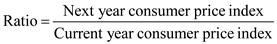# Quiz 8: Purchasing Power Parity and Real Exchange Rates

The consumer index in United States is being provided to determine the inflation in US. Increase in the consumer price index from 350 to 365. Price indexes are nothing but the price level ratios of a given year to the base year price level. Base year remains constant for both the consumer price index under the consideration. In this case, the price indexes ratio has to be determined and subtracting one from the answer will yield the inflation rate.On substituting the variables in the above equation:Thus, the rate of inflation is 4.286%.
Futures trader observes the purchasing and selling price of wheat in Chicago and makes a note of the forward exchange rate between USD and yen for three months. Details are provided in order to determine the transaction costs.First the ratio of future price to forward exchange rate has to be determined in order to determine the forward dollar price.The trader can buy wheat in Chicago for \$3.00 per bushel and sells it in Tokyo, if in case the transportation costs is lesser than the difference that is \$0.14 (i.e. \$3.14 - \$3.00) per bushel, then arbitrage profit is possible. Thus a minimum transaction costs is required to create the situation which do not represent the unexploited profit which is \$0.14 per bushel.